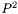#Boost C++ Libraries

...one of the most highly regarded and expertly designed C++ library projects in the world.

This is the documentation for an old version of boost. Click here for the latest Boost documentation.

## Struct template weighted_p_square_cumulative_distribution_impl

boost::accumulators::impl::weighted_p_square_cumulative_distribution_impl — Histogram calculation of the cumulative distribution with thealgorithm for weighted samples.

## Synopsis

```// In header: <boost/accumulators/statistics/weighted_p_square_cumulative_distribution.hpp>

template<typename Sample, typename Weight>
struct weighted_p_square_cumulative_distribution_impl {
// types
typedef numeric::functional::multiplies< Sample, Weight >::result_type            weighted_sample;
typedef numeric::functional::average< weighted_sample, std::size_t >::result_type float_type;
typedef std::vector< std::pair< float_type, float_type > >                        histogram_type;
typedef std::vector< float_type >                                                 array_type;
typedef iterator_range< typename histogram_type::iterator >                       result_type;

// construct/copy/destruct
template<typename Args>
weighted_p_square_cumulative_distribution_impl(Args const &);

// public member functions
template<typename Args> void operator()(Args const &) ;
template<typename Args> result_type result(Args const &) const;
};```

## Description

A histogram of the sample cumulative distribution is computed dynamically without storing samples based on thealgorithm for weighted samples. The returned histogram has a specifiable amount (num_cells) equiprobable (and not equal-sized) cells.

Note that applying importance sampling results in regions to be more and other regions to be less accurately estimated than without importance sampling, i.e., with unweighted samples.

For further details, see

R. Jain and I. Chlamtac, The P^2 algorithmus for dynamic calculation of quantiles and histograms without storing observations, Communications of the ACM, Volume 28 (October), Number 10, 1985, p. 1076-1085.

### `weighted_p_square_cumulative_distribution_impl` public construct/copy/destruct

1. ```template<typename Args>
weighted_p_square_cumulative_distribution_impl(Args const & args);```

### `weighted_p_square_cumulative_distribution_impl` public member functions

1. `template<typename Args> void operator()(Args const & args) ;`
2. `template<typename Args> result_type result(Args const & args) const;`